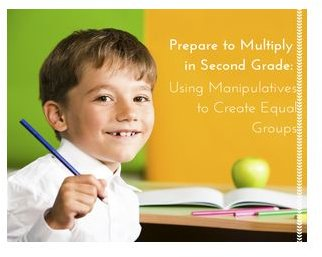# Grade 2 Math Lesson Using Manipulatives to Create Equal Groups – Worksheet Download Included

## ObjectivesDetermine whether a group of objects (up to 20) has an odd or even number of members, e.g., by pairing objects or counting them by 2s; write an equation to express an even number as a sum of two equal addends. 2.OA.C.3.

Use addition to find the total number of objects arranged in rectangular arrays with up to 5 rows and up to 5 columns; write an equation to express the total as a sum of equal addends. 2.OA.C.4.

Materials

## Procedure

Move throughout the classroom placing a handful of counters on each student’s desk. Say, “We have learned about odd and even numbers. I want you to decide if you have an odd or even number of counters. Raise your hand when you are ready to show me how you got your answer.” Move around the room to observe.

Then, “Partner with your neighbor and combine your counters. Now decide if you have an odd or even amount.” Move around the room to observe.

“Now put your counters in groups of two. Do you have any tiles left over? What does it mean if you have a tile left over? (You have an odd number of counters.) Now count by twos to find out how many counters you have.”

“For the next activity please gather 12 counters and put them in front of you. Share your extra counters with those friends who need more counters. Bring any extra counters to me.” Allow time for everyone to have twelve counters.

When ready, “Now I want you to put your counters in two equal groups. Each group will have the same number of counters. How many are in each group? Six.”

Write 6+6=___ on the board. “What is our answer?”

“Now put your counters in four equal groups. How many are in each group? Three.”

Write 3+3+3+3=______. “What is our answer?”

Continue the same process by making three equal groups of chips and six groups of chips. Write an addition equation for each.

“Now we are going to use our counters to make an array. An array is a rectangle. It has rows. Each row has an equal number of objects.”

Demonstrate an array using the board so that all students can see.

X X X X

X X X X

X X X X

Invite the students to make an array with their counters using six counters, then eight and then twelve. How many combinations can they make with each number? Remind them that an array is in the shape of a rectangle and each row has an equal number of objects.

Conclude the lesson by doing the worksheet or assigning it as homework.

Working with arrays as a visual representation of a number will prepare students for the concept of multiplication or repeated addition.

## Extra Activity

Read the book Six-Dinner Sid by Inga Moore. Sid has dinner at six different houses each night. Ask students to make an array of how many dinners Sid would have for a week.

(6 x 7=42) 6+6+6+6+6+6+6= 42 meals

## References

• Kuhns, Catherine and Lasater, Marrie. Common Core Math in Action. Crystal Springs Books, 2013.
• Moore, Inga. Six-Dinner Sid. Aladdin Publishing, 1993.